Adapt models with the Model Compression API

In distributed computing, there are many applications where distributed devices are typically resource-constrained, for example, power, memory, storage, compute, or network. As deep learning and machine learning models become more complex with time, inferencing at distributed network requires model fine-tuning.

Using our data engineer, Meera, she is further tasked with the ability to manage resources that are in various resource-limited environments such as in remote areas where power and network connectivity are constrained. She needs to orchestrate a large number of varying types, from small to midsize servers to small devices and Raspberry Pis that are used in manufacturing plants around the globe. She needs to efficiently compress and deploy some large models in various network nodes automatically. She opts to use the Model Compression API REST services to do the work for her.

In this tutorial, get a step-by-step guide on using the Distributed AI Model Compression API to solve challenges that are associated with fine-tuning models at distributed and edge environments depending on a user’s context.

Following is a complete Python notebook to help you get started using the API. This tutorial also covers a few basic steps such as getting access to a trial subscription of Distributed AI APIs on the IBM API Hub platform.

The tutorial uses a Python notebook, which you can run in your preferred IDE.

Prerequisites

To complete this tutorial, you need:

• An IBM ID
• Python 3.8
• Python notebook IDE

Estimated time

It should take you approximately 30 minutes to complete this tutorial.

Steps

Step 1. Environment setup

1. Navigate to the Distributed AI APIs documentation page, and click Get trial subscription.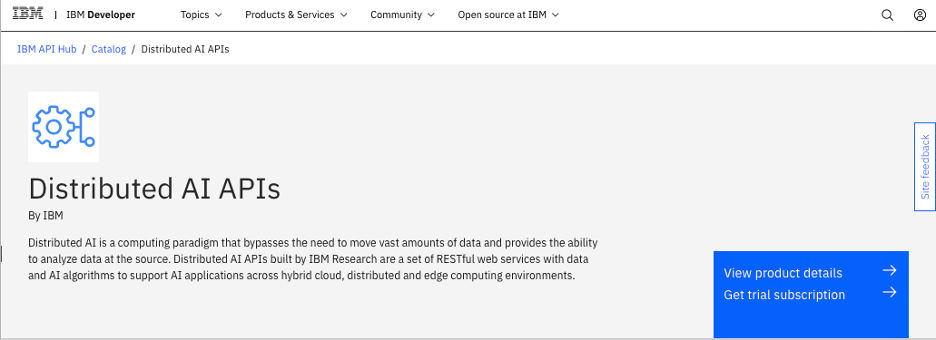2. Log in on the registration page if you already have an IBM ID. Otherwise, create a new IBM ID for yourself.

3. After you log in, the system entitles you with a trial subscription and takes you to the My IBM page. Locate the Trial for Distributed AI APIs tile, and click Launch.

4. On My APIs page, click the Distributed AI APIs tile. When the page opens, locate the Key management section, expand the row to see both the Client ID and Client secret, and click the visibility (eye) icon to reveal the actual values. Make a note of these values because they are the API keys you use throughout this tutorial.5. Create a config.json file with the API key values that you received.

{
"x-ibm-client-id":  "REPLACE_THIS_WITH_YOUR_CLIENT_ID",
"x-ibm-client-secret": "REPLACE_WITH_YOUR_CLIENT_SECRET"
}

6. Install Python package using pip.

pip install sklearn numpy keras tensorflow==2.3 torch==1.8.1 torchvision

Step 2. Invoke the Distributed AI Model Compression API

On the API documentation page, look for the Distributed AI Model Compression API.Step 3. Python notebook example

The example Python notebook provides model pruning for PyTorch and TensorFlow models. Step through the Python notebook code in your preferred Python notebook IDE.

Model Compression API

This notebook showcases the API that performs Model Compression through structured channel pruning for both TensorFlow and PyTorch models. Structured pruning performs a one-shot pruning and returns the model with a user-defined sparcity. Retraining is performed by the user. For more reference information, read Pruning Filters for Efficient ConvNets.

Create a TensorFlow model

# AlexNet
# baseline cnn model from AlexNet
from sklearn.model_selection import KFold

import tensorflow as tf
from tensorflow import keras
from tensorflow.keras.datasets import mnist
from tensorflow.keras.utils import to_categorical
from tensorflow.keras.optimizers import SGD
from tensorflow.python.framework import type_spec as type_spec_module
import os
import numpy as np
import time

from ode.tf_pruner import TfPruner
from ode.tf_quantizer import TfQuantizer

Load, train, and test data set

We define a load_dataset() method as well as a preprocessor in prep_pixels(). We limit the data set to only 1000 elements to keep the training short, and the following statements can be omitted from the following code.

• trainX = trainX[:1000]
• trainY = trainY[:1000]
• testX = testX[:1000]
• testY = testY[:1000]
(trainX, trainY), (testX, testY) = mnist.load_data()

# reshape dataset to have a single channel
trainX = trainX.reshape((trainX.shape, 28, 28, 1))
testX = testX.reshape((testX.shape, 28, 28, 1))

# one hot encode target values
trainY = to_categorical(trainY)
testY = to_categorical(testY)

trainX = trainX[:1000]
trainY = trainY[:1000]
testX = testX[:1000]
testY = testY[:1000]

print(f'trainX.shape: {trainX.shape}')
return trainX, trainY, testX, testY

Defines

# scale pixels
def prep_pixels(train, test):
# convert from integers to floats
train_norm = train.astype('float32')
test_norm = test.astype('float32')

# normalize to range 0-1
train_norm = train_norm / 255.0
test_norm = test_norm / 255.0

# return normalized images
return train_norm, test_norm

Compile the model

def compile_model(model):
opt = SGD(lr=0.01, momentum=0.9)
model.compile(optimizer=opt, loss='categorical_crossentropy', metrics=['accuracy'])

Define the model

def define_model():
"""Model with activation layers"""
model = keras.Sequential() #.to(device=device)
model.add(keras.layers.Conv2D(32, (3, 3), kernel_initializer='he_uniform', input_shape=(28, 28, 1)))

# compile model
compile_model(model)

return model

Initialize the timer, data set, and model

current_milli_time = lambda: int(round(time.time() * 1000))

# prepare cross validation
kfold = KFold(5, shuffle=True, random_state=1)

train_ds_X, train_ds_Y, test_ds_X, test_ds_Y = load_dataset()
train_ds_X, test_ds_X = prep_pixels(train_ds_X, test_ds_X)

# define model
model = define_model()

Train the model

# enumerate splits
for train_ix, test_ix in kfold.split(train_ds_X):

# select rows for train and test
trainX, trainY, testX, testY = train_ds_X[train_ix], train_ds_Y[train_ix], test_ds_X[test_ix], test_ds_Y[test_ix]
# fit model
history = model.fit(trainX, trainY, epochs=10, batch_size=32, validation_data=(testX, testY), verbose=0)
# evaluate model

_, acc = model.evaluate(testX, testY, verbose=0)

print('> %.3f' % (acc * 100.0))

latest_trainX = trainX
latest_trainY = trainY
latest_testX = testX
latest_testY = testY

Evaluate

For evaluation, we keep a subset of the data set in latest_*. Next, we time the prediction for comparison and print the original model stats as well as the summary. There are two ways to access the APIs, either using a command-line through the curl command or through the web interface.

Assumptions

To test the APIs, you must first get your API keys. For reference, see Get trial plan.

curl interface

TensorFlow pruning with curl command at terminal
curl --request POST \
--url 'https://api.ibm.com/edgeai/run/api/tf_prune?percent=REPLACE_THIS_VALUE&ommitted=REPLACE_THIS_VALUE' \
--form model=REPLACE_THIS_VALUE

Note that there are a few values that must be replaced: REPLACE_THIS_VALUE and REPLACE_THIS_KEY, for example. You must first navigate to the directory where you saved the mnist_base.h5 file or the model that you want to prune. You can then invoke the pruning API as follows:

curl --request POST \
--url 'https://api.ibm.com/edgeai/run/api/tf_prune?percent=0.4&ommitted=' \
--form model=@mnist_base.h5

The API parameters are:

• model: This can be either a .zip file that contains the zipped directory saved by using the save() interface or a .h5 file.

• percent: This is the wanted sparcity. For example, 0.4 means to target 40% less channels. So, in theory, the size of the model will be 60% of the original size.

• omitted: This is the list of layers that you want to omit from pruning. Some output layers should fall under this category. In this example, there are no layers. Otherwise, you would include them, separated by commas: for example, ‘fc1,fc2’.

• CLIENT_ID: The client ID obtained when registering to access the APIs.

• CLIENT_SECRET: The client ID secret obtained when registering to access the APIs.

Sample output
{"txid":"cf06e766-2d20-11ec-931e-0242ac170002", "message":"Successfully submitted transaction."}

Save the txid because it is needed to query the API for the operation status. If the upload fails, the txid field is None or N/A, and the message field contains the error message.

Process status

You can obtain the status of each transaction and request through the status API. When the request for pruning is executed, you get a transaction ID (txid) as a result. You can use that txid to check the status of the call itself. The code for the call is:

curl --request GET \
--url 'https://api.ibm.com/edgeai/run/api/status/?txid=REPLACE_THIS_VALUE' \

In this example, the txid was cf06e766-2d20-11ec-931e-0242ac170002, so you invoke the API using the following code.

curl --request GET \
--url 'https://api.ibm.com/edgeai/run/api/status/?txid=cf06e766-2d20-11ec-931e-0242ac170002' \

The API returns the status.

{"status": "0", "message": "Saved model to /tmp/tmp7d8tkpfs.h5", "filename": "tmp7d8tkpfs.h5"}
If the model is either queued or failed, the return will be different. It will have the status field as well as a message field. No filename would be returned in that case.

curl --request GET \
--output 'tmp7d8tkpfs.h5'

% Total    % Received % Xferd  Average Speed   Time    Time     Time  Current
100 2846k  100 2846k    0     0   198M      0 --:--:-- --:--:-- --:--:--  198M

The requirement is the txid.

If the model pruning has failed, this API returns a message with the reason behind the failure as well as the status of the transaction.

{"status":-1,"message":"No transaction submitted for txid"}

Model pruning

Model pruning begins by navigating to tf_prune to upload the model that was saved earlier.

model.save('mnist_base.h5')

Then, the /mnist_base.h5 file is selected from the file system, the desired sparcity percentage is added, and, optionally, the layers to be omitted are passed.

![Model Compression API](https://developer.ibm.com/developer/default/learningpaths/get-started-distributed-ai-apis/model-compression/images/figure4.png)

This creates a response.Save the transaction ID (txid) value from the response field because you use it to check the status.

Pruning status

You can check the status of the pruning request. All requests are asynchronous because model uploading, pruning, and so on, might be time consuming.

Go to the /status page, and enter the txid.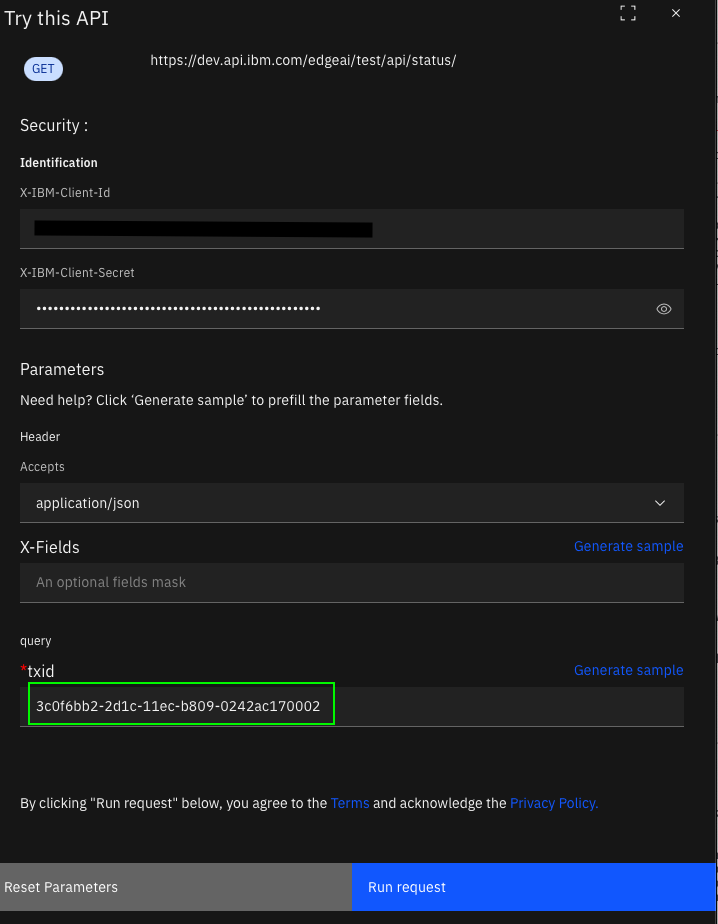You see the response.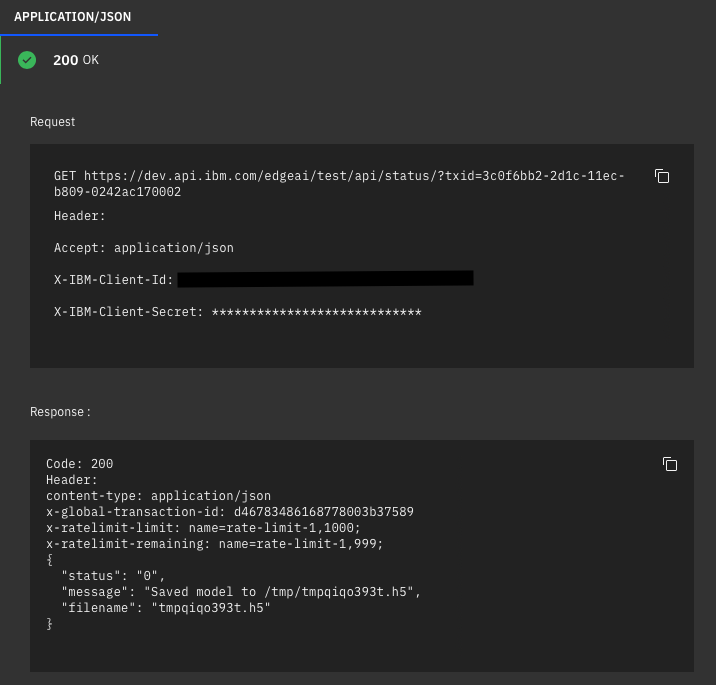Note that the status is 0 (Done). Anything else is accompanied by a message. In this case, models are not downloadable because the system might still be processing requests or the request has failed.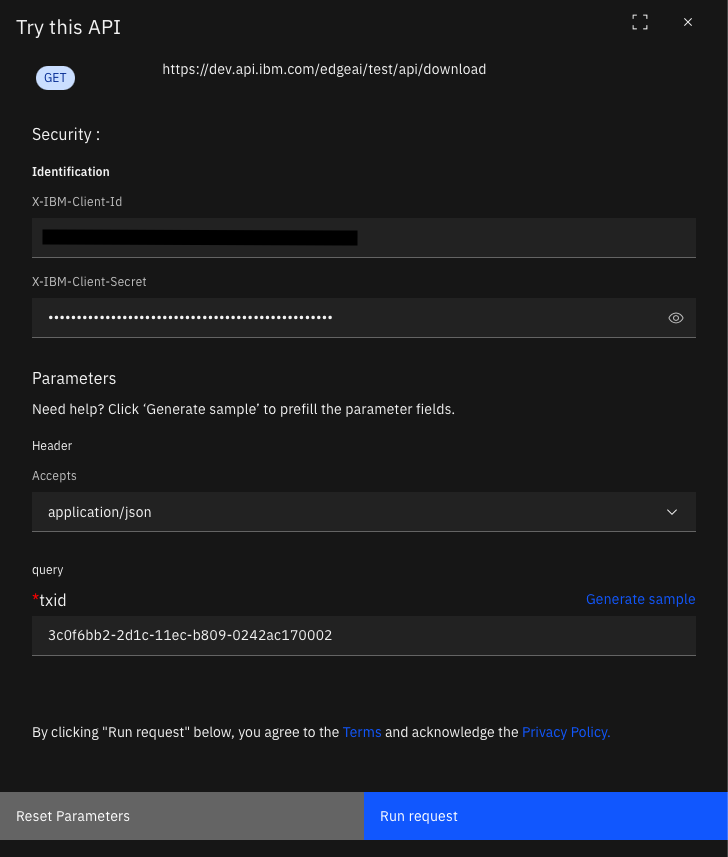You see the response.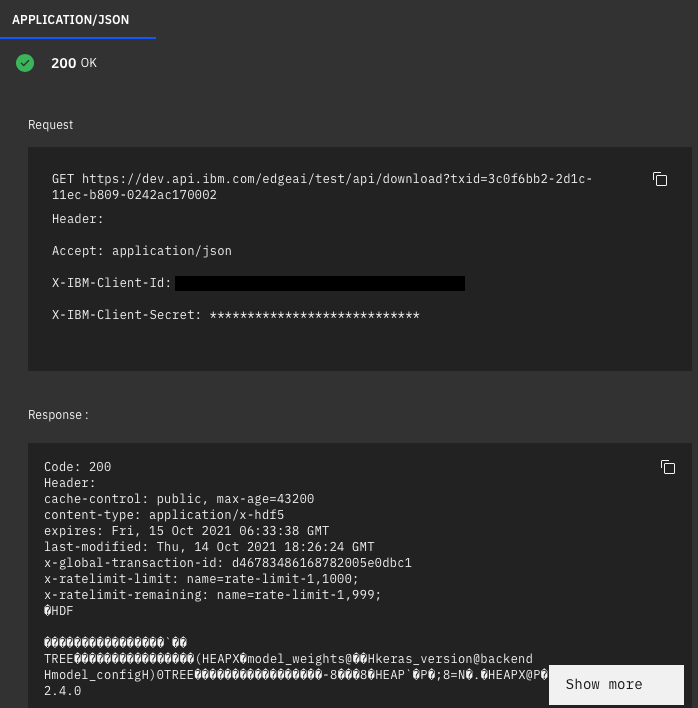Testing pruned model

After the file is downloaded, it is given a temporary file name (for example, tmps34cdcmd.h5). You can then load it by using the model = tf.keras.models.load_model('tmps34cdcmd.h5') API.

The following code shows an example.

pruned_model.summary()

Now, retrain the model to get the accuracy back.

compile_model(pruned_model)

for train_ix, test_ix in kfold.split(train_ds_X):

# select rows for train and test
trainX, trainY, testX, testY = train_ds_X[train_ix], train_ds_Y[train_ix], test_ds_X[test_ix], test_ds_Y[test_ix]
# fit model
history = pruned_model.fit(trainX, trainY, epochs=10, batch_size=32, validation_data=(testX, testY), verbose=0)
# evaluate model

_, acc = pruned_model.evaluate(testX, testY, verbose=0)
latest_testX = testX

print('> %.3f' % (acc * 100.0))

t2 = 0.0
for i in range(0, len(latest_testX)):

img = latest_testX[i]
img = (np.expand_dims(img,0))

t1 = current_milli_time()
prediction = pruned_model.predict(img)
t2 += current_milli_time() - t1

t2 /= float(len(latest_testX))

print('> Pruned Model Accuracy: %.3f' % (acc * 100.0))
print('> Pruned Model Inference Time: {}'.format(t2))

pruned_model.save('mnist_pruned.h5')

Create PyTorch models

PyTorch has some limitations when saving the full model. However, this is more of a pickle issue than PyTorch. PyTorch relies on pickle for serialization. Therefore, it suffers from the same issue. For now, you cannot save full PyTorch models and upload them directly to the cloud. This is because when the model is loaded it looks for class names and other environment-specific metadata that is only present in the developer’s machine. This means that we require PyTorch models to upload two files for the model: the model definition and the model weights (state dictionary).

First, we define the model and all other helpers.

from __future__ import print_function
import argparse
import torch
import torch.nn as nn
import torch.nn.functional as F
import torch.optim as optim
from torchvision import datasets, transforms
from torch.optim.lr_scheduler import StepLR

class Net(nn.Module):
def __init__(self):
super(Net, self).__init__()
self.conv1 = nn.Conv2d(1, 32, 3, 1)
self.conv2 = nn.Conv2d(32, 64, 3, 1)
self.dropout1 = nn.Dropout(0.25)
self.dropout2 = nn.Dropout(0.5)
self.fc1 = nn.Linear(9216, 128)
self.fc2 = nn.Linear(128, 10)

def forward(self, x):
x = self.conv1(x)
x = F.relu(x)
x = self.conv2(x)
x = F.relu(x)
x = F.max_pool2d(x, 2)
x = self.dropout1(x)
x = torch.flatten(x, 1)
x = self.fc1(x)
x = F.relu(x)
x = self.dropout2(x)
x = self.fc2(x)
output = F.log_softmax(x, dim=1)
return output

def train(args, model, device, train_loader, optimizer, epoch):
model.train()
for batch_idx, (data, target) in enumerate(train_loader):
data, target = data.to(device), target.to(device)
output = model(data)
loss = F.nll_loss(output, target)
loss.backward()
optimizer.step()
if batch_idx % args.log_interval == 0:
print('Train Epoch: {} [{}/{} ({:.0f}%)]\tLoss: {:.6f}'.format(
100. * batch_idx / len(train_loader), loss.item()))
if args.dry_run:
break

model.eval()
test_loss = 0
correct = 0
data, target = data.to(device), target.to(device)
output = model(data)
test_loss += F.nll_loss(output, target, reduction='sum').item()  # sum up batch loss
pred = output.argmax(dim=1, keepdim=True)  # get the index of the max log-probability
correct += pred.eq(target.view_as(pred)).sum().item()

print('\nTest set: Average loss: {:.4f}, Accuracy: {}/{} ({:.0f}%)\n'.format(

Next, we initialize, build, and train the model.

use_cuda = not args.no_cuda and torch.cuda.is_available()

torch.manual_seed(args.seed)

device = torch.device("cuda" if use_cuda else "cpu")

train_kwargs = {'batch_size': args.batch_size}
test_kwargs = {'batch_size': args.test_batch_size}
if use_cuda:
cuda_kwargs = {'num_workers': 1,
'pin_memory': True,
'shuffle': True}
train_kwargs.update(cuda_kwargs)
test_kwargs.update(cuda_kwargs)

transform=transforms.Compose([
transforms.ToTensor(),
transforms.Normalize((0.1307,), (0.3081,))
])
transform=transform)
dataset2 = datasets.MNIST('../data', train=False,
transform=transform)

model = Net().to(device)

scheduler = StepLR(optimizer, step_size=1, gamma=args.gamma)
for epoch in range(1, args.epochs + 1):
train(args, model, device, train_loader, optimizer, epoch)
scheduler.step()

#if args.save_model:
torch.save(model.state_dict(), "mnist_cnn.pth")

Now, we can either use the RESTful interface or the web interface similar to the TensorFlow implementation.

PyTorch pruning

For PyTorch, you need a few extra parameters because you cannot directly load the module and run the model. This is because of the limitations in pickle.

To invoke the RESTful API, you must specify the following fields:

• weights: This is the state dictionary. For example:

torch.save(model.state_dict(), "mnist_cnn.pth")

• dataset: This is a sample data set that is generated by saving the data set using the torch.save() method. For example:

• class_def: This is the Python file with all of the necessary libraries that are needed to build the model. This must be a stand-alone Python file. The only libraries that we support are the base Torch library. The following code shows an example.

import torch
import torch.nn as nn
import torch.nn.functional as F

class Net(nn.Module):
def __init__(self):
super(Net, self).__init__()
self.conv1 = nn.Conv2d(1, 32, 3, 1)
self.conv2 = nn.Conv2d(32, 64, 3, 1)
self.dropout1 = nn.Dropout(0.25)
self.dropout2 = nn.Dropout(0.5)
self.fc1 = nn.Linear(9216, 128)
self.fc2 = nn.Linear(128, 10)

def forward(self, x):
x = self.conv1(x)
x = F.relu(x)
x = self.conv2(x)
x = F.relu(x)
x = F.max_pool2d(x, 2)
x = self.dropout1(x)
x = torch.flatten(x, 1)
x = self.fc1(x)
x = F.relu(x)
x = self.dropout2(x)
x = self.fc2(x)
output = F.log_softmax(x, dim=1)
return output
• model_name: This is the main class of the model. We assume that the model is created through a generic constructor with no parameters (for example, model = Net()).

• percent: This is the desired sparcity. For example, 0.4 means to target 40% less channels. In theory, the size of the model is 60% of the original size.

• omitted: This is the list of layers that you want to omit from pruning. Most output layers should fall under this category. For example, you might want to omit fc1 and fc2 in the previous code, especially fc2 because it is the main output layer.

• input_size: This is the input size of the model input. It can be obtained by getting an inference instance and looking at the shape of the tensor or numpy array. For example, a single 28×28 image would be 1,28,28.

The API can be invoked by using the following code at your terminal.

curl --request POST \
--url 'https://api.ibm.com/edgeai/run/api/pt_prune?percent=0.5&ommitted=fc1,fc2&input_size=1,28,28&model_name=Net' \
--form weights=@mnist_cnn.pth \
--form class_def=@model.py

Note that in the previous example, all of the files are in the same directory. However, you can specify the path to your file manually.

The response from this call is:

{"txid": "bbab7210-2d1e-11ec-917d-0242ac170002", "message": "Successfully submitted transaction."}
Process status

You can obtain the status of each transaction and request through the status API. When the request for pruning is executed, you get a transaction ID (txid) as a result. You can use that txid to check the status of the call itself. The code for the call is:

curl --request GET \
--url 'https://api.ibm.com/edgeai/run/api/status/?txid=bbab7210-2d1e-11ec-917d-0242ac170002' \

The API returns the status.

{"status": "0", "message": "b'----------------------------------------------------------------\\n        Layer (type)               Output Shape         Param #\\n================================================================\\n            Conv2d-1           [-1, 16, 26, 26]             160\\n            Conv2d-2           [-1, 32, 24, 24]           4,640\\n           Dropout-3           [-1, 32, 12, 12]               0\\n            Linear-4                  [-1, 128]         589,952\\n           Dropout-5                  [-1, 128]               0\\n            Linear-6                   [-1, 10]           1,290\\n================================================================\\nTotal params: 596,042\\nTrainable params: 596,042\\nNon-trainable params: 0\\n----------------------------------------------------------------\\nInput size (MB): 0.00\\nForward/backward pass size (MB): 0.26\\nParams size (MB): 2.27\\nEstimated Total Size (MB): 2.54\\n----------------------------------------------------------------\\n'", "filename": "tmpqpj73q22.pt"}

If the model is either queued or failed, the return is different. It will have the status field as well as a message field. In this case, no file name is returned. In this example, the message consists of the model summary.

curl --request GET \
--output 'tmpqpj73q22.pt'

% Total    % Received % Xferd  Average Speed   Time    Time     Time  Current
100 2846k  100 2846k    0     0   198M      0 --:--:-- --:--:-- --:--:--  198M

The requirement is the txid. The wanted file name is passed through the --output flag, as shown in the previous code. The status API returns the name of the model because it was generated by the pruning APIs.

PyTorch prune interface

To test the PyTorch pruning API, navigate to the /pt_prune endpoint, and complete all of the necessary fields.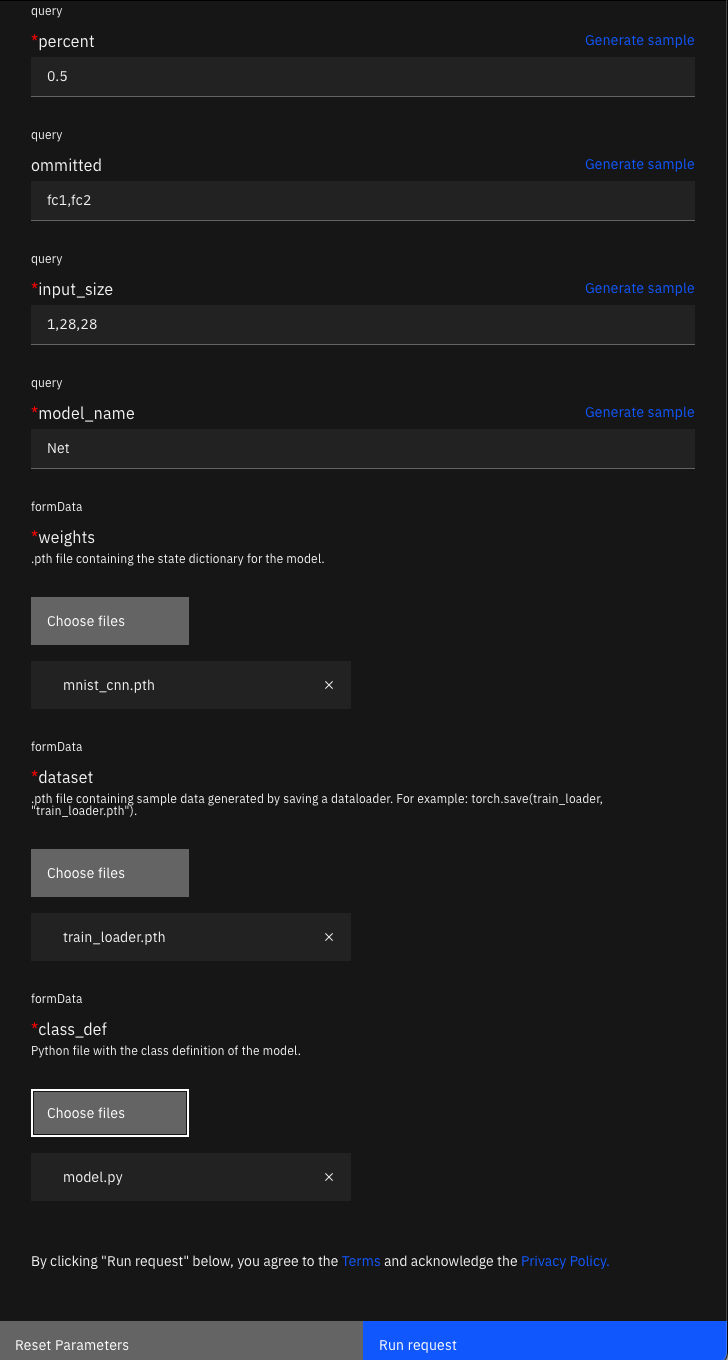This gives you a response that looks like the example in the following image.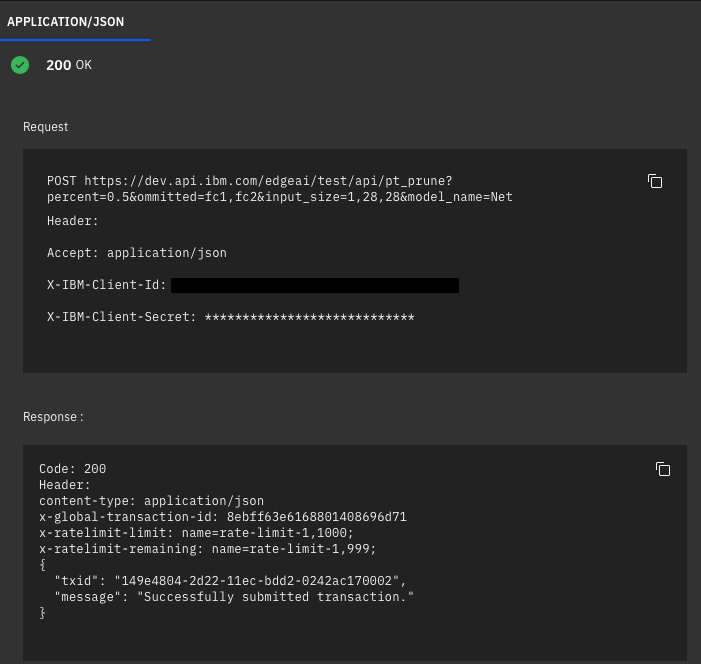The next step is to check the status and download the model. These steps are the same as the TensorFlow instance.

PyTorch prune status interface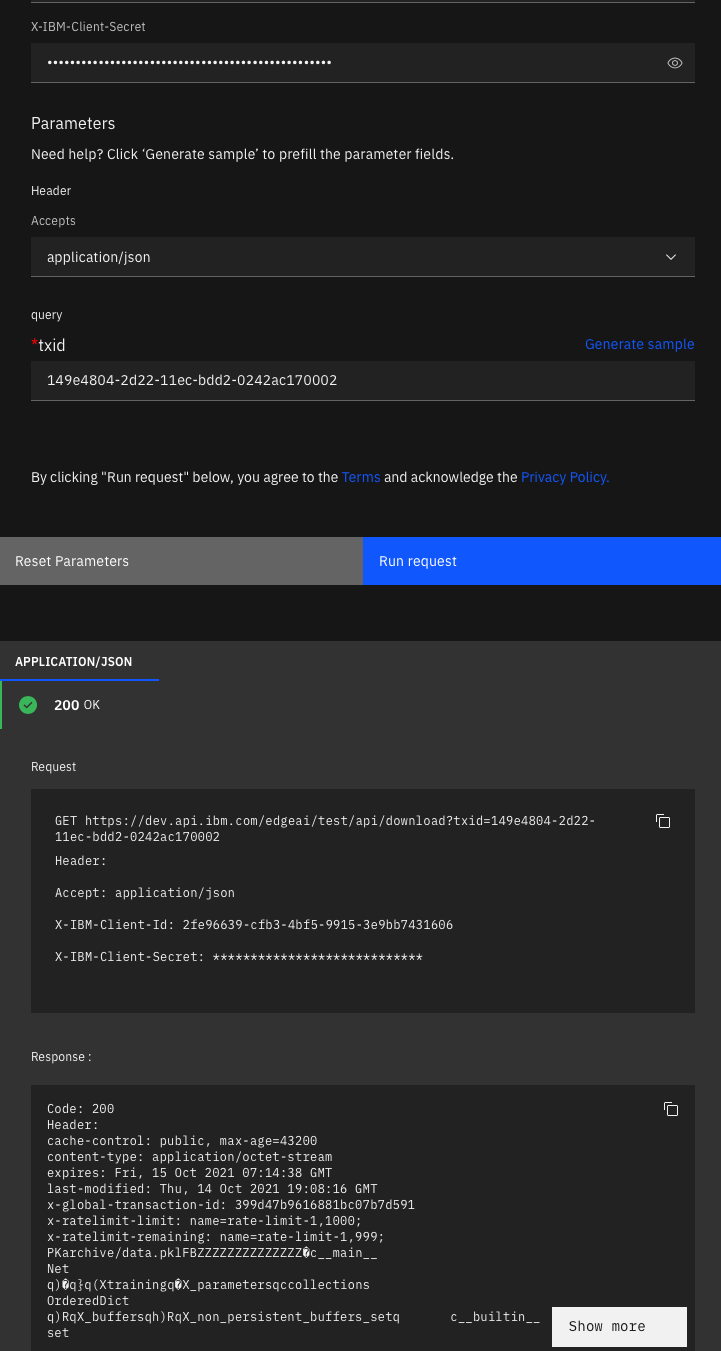Testing the model

use_cuda = not args.no_cuda and torch.cuda.is_available()
device = torch.device("cuda" if use_cuda else "cpu")

model.eval()

Now, you can retrain the model using your standard training loop, for example:

scheduler = StepLR(optimizer, step_size=1, gamma=args.gamma)
for epoch in range(1, args.epochs + 1):
train(args, model, device, train_loader, optimizer, epoch)